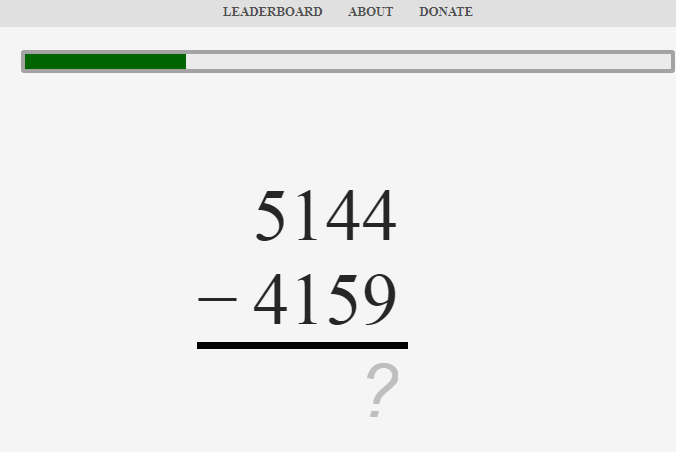# Mental calculation is memorization

I have discovered that mental calculation is actually memorization, so tell me how do all of you memorize numbers while calculating in your mind.

1 Like

If you do 57 + 97, how and what are you memorizing?

I see all the numbers in my mind like you would on a piece of paper.

Example: 34×57

First I multiply 57 by 30. 57 becomes 570, 500 times 3 is 1500, 1500 is for now the answer. 70 times 3 is 210. I then add 210 to 1500, which becomes 1710. Next up is 4×57. First I calculated 4×50 which is 200, I add that to 1710, which turns into 1910. Now I only need to multiply 4 with 7 and add that to 1910 and I arrive at the answer of 1938.

1 Like

57+97 = ?
memory that 7+7 = 14
memory that 4+0 = 4
memory that 1+5 = 6
memory that 6+9 = 15
-> 57+97 = 154
Or by any larger chunks.
What if you did not have any sort of memory and were calculating it how would this differ ?
You can’t do 50+90 = 140, you have to work in chunks that you know or have in memory which you can’t for this example. If you do not have chunks in memory you require counting assuming you have at-least sufficient general memory for counting.
97 +1
57 - 1
98 +1
56 -1

Hence it is valid that mental calculation is actually memorization, at-least to some extent…I think its quite a lot more about using your memory as well, but I assume this is what the OP meant in a way. If not I highlighted this point at least.

Simply said, the only reason you know or can work out things like 7*4 is because you have the memory of the tables of either 4 or 7, know the answer or any smaller chunk thereof.
Otherwise you would be counting fingers or similar.

1 Like

I do indeed not use mnemonics for memorizing numbers (atleast when calculating), I tend to find that if I calculate quickly the numbers stay even if large. So I kind of have a visual sight in my head of the numbers and rapidly update them. If I can’t do the sum quickly enough to not lose track of the numbers perhaps if it is large, I take more time and this is when I may accommodate using my pegs for whatever numbers I can to reduce the load.

I do use some methods sort of though.
Multiplication I do from right to left for example
38*21

8* 1 , (2* 8+3* 1) ,(3* 2)
8,19,6
=798
Addition in columns right to left again
22+81
1+2 =3
8+2 = 10
=103

1 Like

Im doing
5 + 9 = 14;
147 + 7 = 154

I am working out on math trainer but I’ve perceived that I was looking the number of counts and it becomes easier like this. Im triying to do it seeing the questions quickly, saving them in my mind and solving in my mind. It’s hard to me save addition subtracting numbers of 4 algarisms either parcial results.There is a trade off between memory and processing speed. The more facts you know or computation tricks you know (fluently) the less you have spend thinking. In computer programming we often play with this balance - lookup tables vs calculation.

But realize that your brain does a lot of thinking and processing that you are not aware of. Speaking English is very complicated process but usually you are not aware of forming the sentences. Sometimes calculation results just appear in my head fully formed. Sometimes I’m skeptical, a wild guess perhaps, but yep it was right.

If you play a sport it will often seem as if your body is doing the thinking. Movement patterns emerge spontaneously and combine to form coherent sequences just like speaking.

1 Like